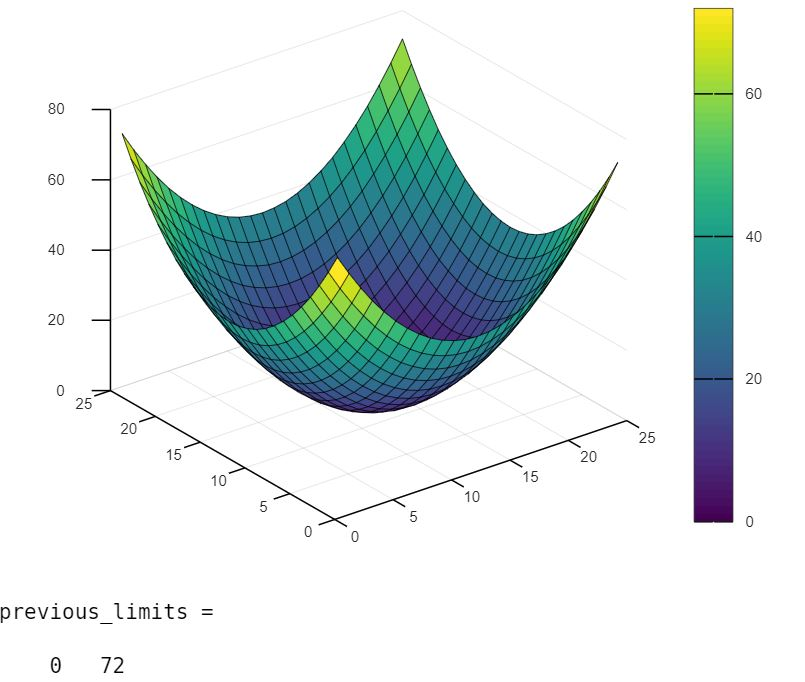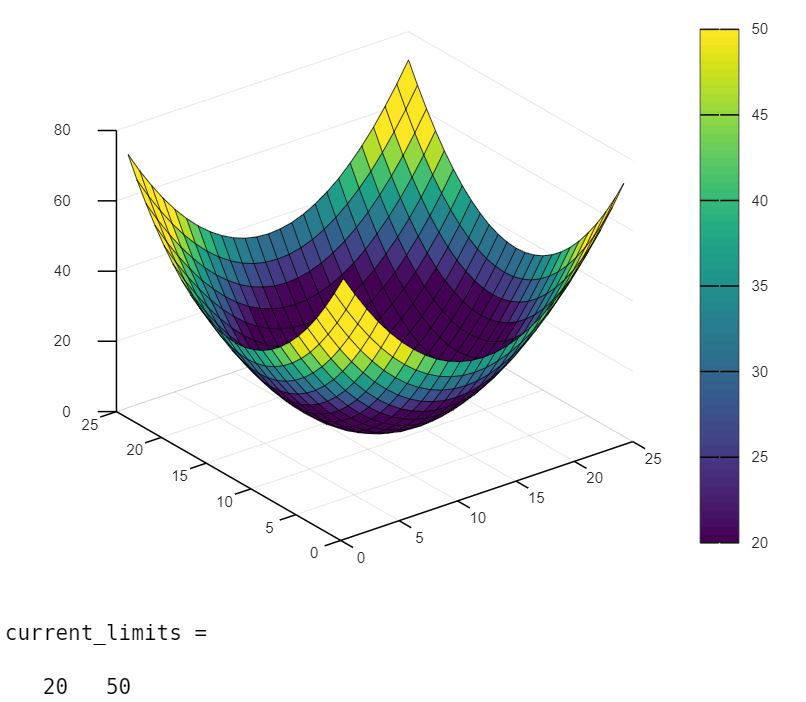# The Caxis() Function in MATLAB

This tutorial will discuss setting the colormap limits using the `caxis()` or `clim()` function in MATLAB.

## Use the `caxis()` Function in MATLAB

The `caxis()` function, which is renamed to `clim()` function in MATLAB’s version R2022a, is used to set the limits of the colormap in different plots. We can use the `caxis()` function if the MATLAB version is older than the version R2022a and if the version is R2022a or newer, we have to use the `clim()` function.

The `caxis()` or `clim()` function have five syntaxes which are given below.

Syntax:

``````clim(axis_limits)
clim("auto")
clim("manual")
clim(target_axis,___)
lims_object = clim
``````

The `clim(axis_limits)` sets the limits of the colormap according to the limits present in the `axis_limits` variable. The `axis_limits` variable should be a two-element vector, where the first value defines the minimum limit and the second value defines the maximum limit.

If there are values in the default colormap which are less than or equal to the minimum limit of the new colormap limits, then the previous values will be mapped to the first row of the new colormap. If there are values in the default colormap which are greater than or equal to the maximum limit of the new colormap limits, then the previous values will be mapped to the last row of the new colormap.

The `clim("auto")` is the default behavior of the function, which will set the colormap limits according to the indexes of the plot. We can also use the `clim auto` or `caxis auto` command instead of the above syntax to set the limits to auto.

The `clim("manual")` will turn off the automatic limit updates. We can also use the `clim manual` or `caxis manual` command instead of the above syntax to disable automatic limit updates.

The`clim(target_axis,___)` is used to specify the axis whose colormap values we want to change. This syntax is useful if we don’t want to change the colormap limits of all the colormaps present in the current plot.

The `lims_object = clim` will return the colormap limits of the current colormap. For example, let’s change the default colormap limits of a surface plot.

Example Code:

``````[x_axis,y_axis] = meshgrid(-6:.5:6);
z_axis = x_axis.^2 + y_axis.^2;
surf(z_axis);
colorbar
previous_limits = caxis
caxis([20 50])
current_limits = caxis
``````

Output:We used the `meshgrid()` function to create a grid for the surface plot. We use the `surf()` function to create the surface plot and the `colorbar` command to create a bar of colors that will show the value for the corresponding color present in the plot.

The output shows that the previous colormap limits are `0 to 72` and the new limits are `20 to 50`. Check this article for more details about the `caxis()` or `clim()` function.

Write for us
DelftStack articles are written by software geeks like you. If you also would like to contribute to DelftStack by writing paid articles, you can check the write for us page.

## Related Article - MATLAB Plot

• MATLAB Polar Plot
• Plot Multiple Data Sets on a Single Scatter Plot in MATLAB
• Use Greek Symbols in Bar Graph Labels in MATLAB